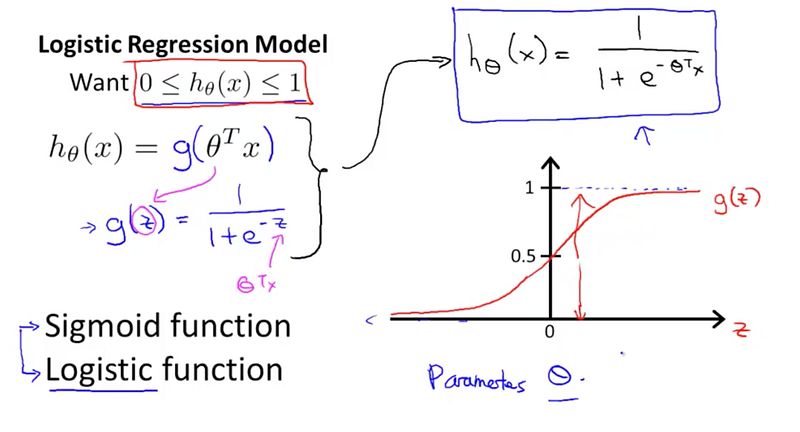# Unraveling Logistic Regression: An Essential Guide for Predictive Analysis### Introduction

Logistic regression, much like linear regression, stands as a fundamental method in predictive analytics. However, while linear regression is typically employed for predicting quantitative outputs, logistic regression shines in the realm of categorical predictions, primarily binary. This comprehensive article dives into the concept of logistic regression, demystifying its principles, applications, and nuances within the predictive analytics landscape.

### Understanding Logistic Regression: The Basics

Logistic regression is a statistical model used for binary classification problems. Essentially, it estimates the probability that an instance belongs to a particular class. If the estimated probability is greater than 50%, the model predicts that the instance belongs to that class (referred to as the positive class, labeled “1”), and otherwise, it predicts that it does not (i.e., it belongs to the negative class, labeled “0”).

#### The Logistic Function

Unlike linear regression that can output any real-number value, logistic regression uses the logistic function (also known as the sigmoid function) to ensure that the output remains between 0 and 1. This characteristic makes it a suitable choice for probability estimation and binary classification. The logistic function is given by:

p = 1 / (1 + e^(-z))

Here,
– p is the probability of the positive class,
– e is the base of natural logarithms,
– z is a linear combination of input features (z = b0 + b1*x1 + b2*x2 + … + bn*xn).

### Logistic Regression for Binary Classification

Logistic regression is commonly used for binary classification problems, such as determining whether an email is spam or not spam, or whether a tumor is malignant or benign. The output of a logistic regression model is a probability that the given input point belongs to a certain class. The threshold at 0.5 is usually used for decision making. If the probability is greater than 0.5, the data is labeled 1. Less than 0.5, it is labeled 0.

### Logistic Regression for Multiclass Classification

Although traditionally used for binary classification, logistic regression can be extended to handle multiclass classification problems — scenarios where the target variable has more than two possible outcomes. This extension is often referred to as “softmax regression” or “multinomial logistic regression”.

### Assumptions in Logistic Regression

Much like other statistical models, logistic regression operates under certain assumptions. These include:

1. Independence of Observations: Each observation should be independent of one another.
2. Linearity of independent variables and log odds: Although logistic regression predicts a non-linear outcome (probability), the logistic regression algorithm assumes a linear relationship between the independent variables and the log-odds of the dependent variable.
3. Absence of multicollinearity: The predictor variables should not be highly correlated with each other, which is a problem known as multicollinearity.
4. Large Sample Size: Logistic regression is better suited for situations where the dataset is sufficiently large. Small sample sizes can lead to overfitting.

### Applications of Logistic Regression

Despite its age and relatively simple nature, logistic regression is highly versatile and continues to find widespread use across a range of sectors:

– In healthcare, logistic regression can be used to predict whether a patient has a given disease (based on symptoms and personal information).
– In marketing, it can predict whether a customer will buy a product or churn.
– In finance, it can predict whether a borrower will default on a loan.
– In machine learning, it can categorize texts based on sentiment (positive, neutral, negative), or identify whether a transaction is fraudulent.

### Logistic Regression in Machine Learning

Logistic regression holds an important place in the field of machine learning. Despite being a statistical technique, its application in predicting categorical outcomes has led to its acceptance in the machine learning world. It is often used as a baseline model for binary classification problems. Its simplicity, interpretability, and efficient computation even on large datasets are the keys to its wide usage.

### Wrapping Up

In conclusion, logistic regression is a powerful yet straightforward analytical tool for binary and multiclass classification problems. Its principle of employing the logistic function to model the data provides the base for many machine learning algorithms, thus cementing its place in every data scientist’s toolbox. Its widespread applications across various sectors further emphasize the importance of understanding and applying logistic regression in data analysis.

# Personal Career & Learning Guide for Data Analyst, Data Engineer and Data Scientist

## Applied Machine Learning & Data Science Projects and Coding Recipes for Beginners

A list of FREE programming examples together with eTutorials & eBooks @ SETScholars

# Projects and Coding Recipes, eTutorials and eBooks: The best All-in-One resources for Data Analyst, Data Scientist, Machine Learning Engineer and Software Developer

Topics included:Classification, Clustering, Regression, Forecasting, Algorithms, Data Structures, Data Analytics & Data Science, Deep Learning, Machine Learning, Programming Languages and Software Tools & Packages.
(Discount is valid for limited time only)## Find more … …

Machine Learning Project – Logistic Regression in Machine Learning with Python

Statistics with R for Business Analysts – Logistic Regression

Machine Learning for Beginners in Python: Logistic Regression On Very Large Data